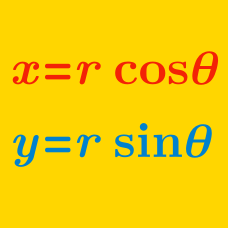Algebra

# Polar Coordinates - Convert Functions

The line $y = ax + b$ in Cartesian coordinates can be written as $r = \frac{13}{\sin \theta - 24 \cos \theta}$ in polar coordinates. Assuming $b$ is positive, what is the value of $a + b$?

The graph $r= \frac{2}{1-6\sin \theta}$ in polar coordinates can be expressed as $x^2 = ay^2+by+c$ in Cartesian coordinates, where $a$, $b$ and $c$ are real numbers. What is the value of $a+b+c$?

Letting $\theta$ vary from $0$ to $2\pi,$ the parametric equations $x= 5 + \cos \theta$ and $y = \sin \theta$ represent a circle $\Gamma_1.$ There is a circle $\Gamma_2$ that is externally tangent to $\Gamma_1,$ tangent to the $y$-axis, and centered (in Cartesian coordinates) at $(10, \sqrt{a}).$ What is the value of $a?$

If $x$ and $y$ satisfy $x=3 \cos \theta$ and $y = 8 + 3 \sin \theta$, the graph of $(x, y)$ is a circle. If the center of this circle is $(a, b)$ and the radius is $r$, what is the value of $a+b+r$?

Which of the following Cartesian coordinate equations represents the polar equation $r=14 \cos \theta\ (0 \leq \theta < 2\pi)?$

×